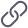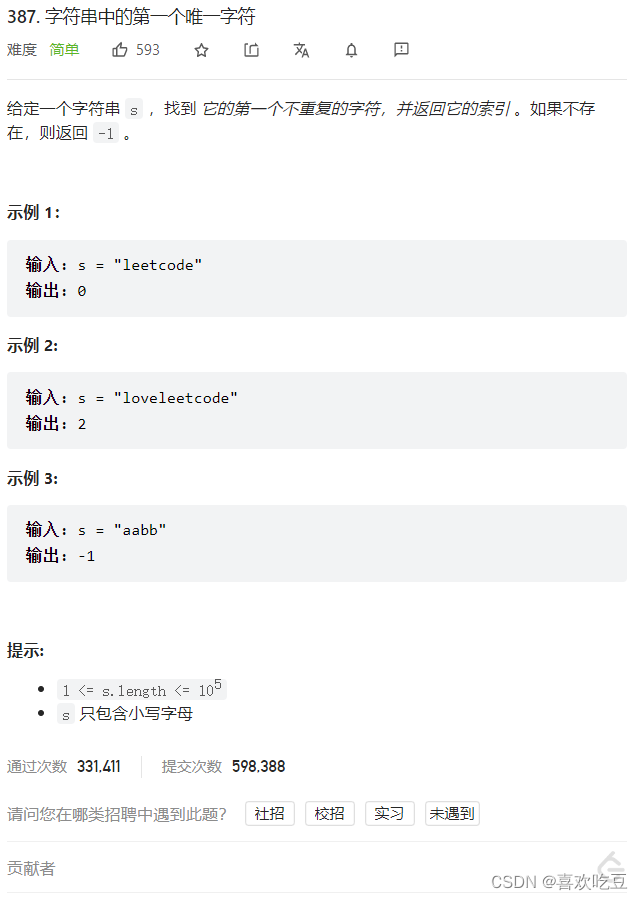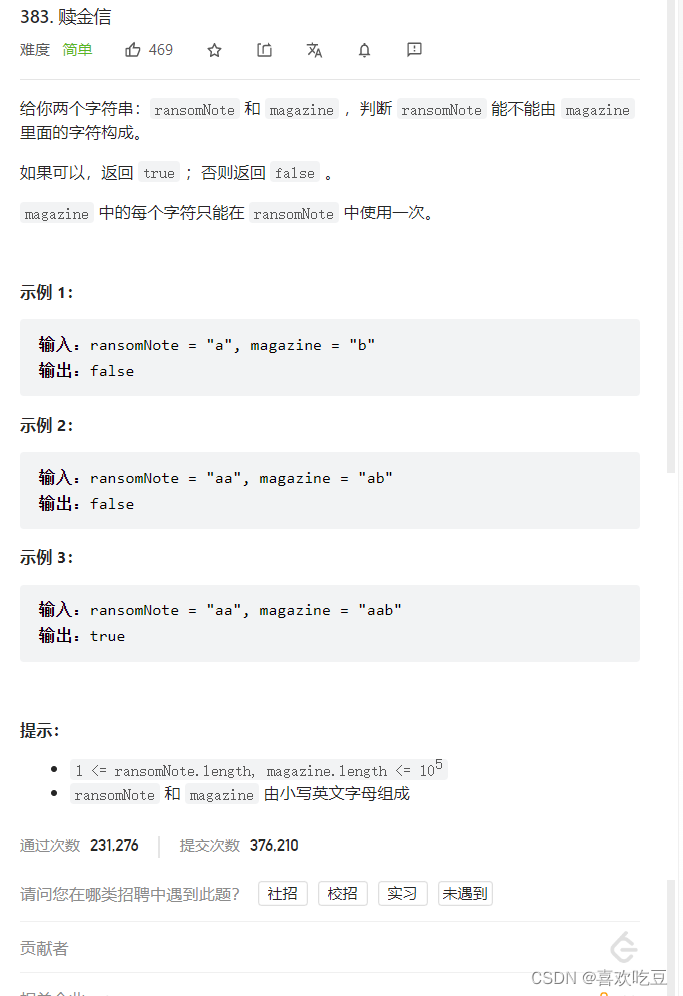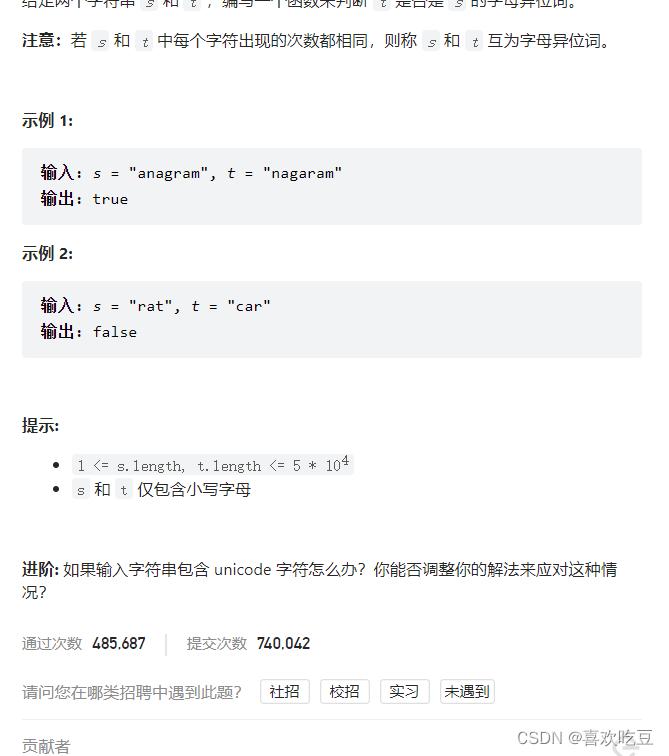1，使用哈希表存储频数

2，使用哈希表存储索引

3，队列

1，字符统计

​编辑1，排序

2，哈希表

# 一，字符串中的第一个唯一字符

387. 字符串中的第一个唯一字符 - 力扣（LeetCode）https://leetcode.cn/problems/first-unique-character-in-a-string/?plan=data-structures&plan_progress=ggfacv7## 1，使用哈希表存储频数

### 思路与算法

class Solution {
public:
int firstUniqChar(string s) {
unordered_map<int, int> frequency;
for (char ch: s) {
++frequency[ch];
}
for (int i = 0; i < s.size(); ++i) {
if (frequency[s[i]] == 1) {
return i;
}
}
return -1;
}
};



## 2，使用哈希表存储索引

### 思路与算法

class Solution {
public:
int firstUniqChar(string s) {
unordered_map<int, int> position;
int n = s.size();
for (int i = 0; i < n; ++i) {
if (position.count(s[i])) {
position[s[i]] = -1;
}
else {
position[s[i]] = i;
}
}
int first = n;
for (auto [_, pos]: position) {
if (pos != -1 && pos < first) {
first = pos;
}
}
if (first == n) {
first = -1;
}
return first;
}
};



## 3，队列

### 小贴士

class Solution {
public:
int firstUniqChar(string s) {
unordered_map<char, int> position;
queue<pair<char, int>> q;
int n = s.size();
for (int i = 0; i < n; ++i) {
if (!position.count(s[i])) {
position[s[i]] = i;
q.emplace(s[i], i);
}
else {
position[s[i]] = -1;
while (!q.empty() && position[q.front().first] == -1) {
q.pop();
}
}
}
return q.empty() ? -1 : q.front().second;
}
};



# 二，赎金信

383. 赎金信 - 力扣（LeetCode）https://leetcode.cn/problems/ransom-note/## 1，字符统计

●如果字符串magazine 的长度小于字符串ransomNote的长度，则我们可以肯定magazine无法构成ransomNote,此时直接返回false。
●首先统计magazine; 中每个英文字母a的次数cnt[a]，再遍历统计ransomNote中每个英文字母

class Solution {
public:
bool canConstruct(string ransomNote, string magazine) {
if (ransomNote.size() > magazine.size()) {
return false;
}
vector<int> cnt(26);
for (auto & c : magazine) {
cnt[c - 'a']++;
}
for (auto & c : ransomNote) {
cnt[c - 'a']--;
if (cnt[c - 'a'] < 0) {
return false;
}
}
return true;
}
};



### 复杂度分析

●时间复杂度: O(m+n)，其中m是字符串ransomNote的长度，n是字符串
magazine的长度，我们只需要遍历两个字符一-次即可。
●空间复杂度: O(|S|)， S是字符集，这道题中S为全部小写英语字母，因此|S|= 26。

# 三，有效的字母异位词

242. 有效的字母异位词 - 力扣（LeetCode）https://leetcode.cn/problems/valid-anagram/?plan=data-structures&plan_progress=ggfacv7

##1，排序

t 是 s 的异位词等价于「两个字符串排序后相等」。因此我们可以对字符串 s 和 t 分别排序，看排序后的字符串是否相等即可判断。此外，如果 s 和 t 的长度不同，t 必然不是 s 的异位词。

class Solution {
public:
bool isAnagram(string s, string t) {
if (s.length() != t.length()) {
return false;
}
sort(s.begin(), s.end());
sort(t.begin(), t.end());
return s == t;
}
};



### 复杂度分析

●时间复杂度: O(nlogn), 其中n为s的长度。排序的时间复杂度为O(n logn),比较两个字符串是否相等时间复杂度为O(n),因此总体时间复杂度为O(nlogn+ n) = O(n logn)。
●空间复杂度: O(logn)。 排序需要O(logn)的空间复杂度。注意，在某些语言(比如Java&JavaScript)中字符串是不可变的，因此我们需要额外的O(n)的空间来拷贝字符串。但是我们

。这依赖于语言的细节;
。这取决于函数的设计方式，例如，可以将函数参数类型更改为char[] 。

## 2，哈希表

class Solution {
public:
bool isAnagram(string s, string t) {
if (s.length() != t.length()) {
return false;
}
vector<int> table(26, 0);
for (auto& ch: s) {
table[ch - 'a']++;
}
for (auto& ch: t) {
table[ch - 'a']--;
if (table[ch - 'a'] < 0) {
return false;
}
}
return true;
}
};



### 复杂度分析

• 时间复杂度：O(n)O(n)，其中 n 为 s 的长度。

• 空间复杂度：O(S)，其中 S 为字符集大小，此处S=26。

class Solution {
public:
bool isAnagram(string s, string t) {
int freq {};
for (char ch : s) ++freq[ch - 'a'];
for (char ch : t) --freq[ch - 'a'];
return all_of(begin(freq), end(freq), [](int x) { return x == 0; });
}
};

08-10879
02-1218
01-27137
12-0363
07-30249
08-17102
12-25206
05-202764
09-133232
08-14145
02-29547
08-0541
07-2494
02-2383
02-151406
10-286458
01-17739
01-27322

### “相关推荐”对你有帮助么？

•非常没帮助
•没帮助
•一般
•有帮助
•非常有帮助被折叠的  条评论 为什么被折叠?到【灌水乐园】发言¥2 ¥4 ¥6 ¥10 ¥20余额支付 (余额：-- )扫码支付获取中扫码支付点击重新获取扫码支付1.余额是钱包充值的虚拟货币，按照1:1的比例进行支付金额的抵扣。
2.余额无法直接购买下载，可以购买VIP、C币套餐、付费专栏及课程。余额充值